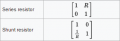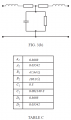# High voltage transmission line with ABCD complex equations

#### bizuputyi

Joined Aug 3, 2014
21
Figure shows a 50 Hz, high-voltage, transmission line. The relationships between the sending and receiving end voltages and currents are given by the complex ABCD equations:

$$V_S=V_R(A_1+jA_2)+I_R(B_1+jB_2)$$

$$I_S=V_R(C_1+jC_2)+I_R(D_1+jD_2)$$

where 'S' stands for sending-end and 'R' stands for receiving-end

(a) Given the parameter values in TABLE C and an open-circuit received voltage measured as 88.9 kV, calculate the values of $$V_S$$ and $$I_S$$ and hence the power $$P_{SO}$$ absorbed from the supply by the transmission line on open circuit.

(b) If the line is modelled by the T-circuit of FIGURE 3(b), see if you can estimate the primary line coefficients R, L, G and C. The line is 50 km long.

What I'm thinking is $$I_R=0$$ as this is an open-circuit and given $$V_R$$; $$V_S$$ and $$I_S$$ can be calculated with matrix.

$$V_S=77325+j3149 KV$$
$$I_S=j119.9A$$

But I don't think that is correct because $$V_S$$ should not be lower than $$V_R$$, also 50Hz frequency is given for a reason, I'm sure it has to be used somewhere.

And as for question (b) I have only got ideas. I can find coefficient of propagation γ and $$Zo$$ from R,L,G,C but I don't know how to produce them vice versa (from ABCD), I don't see where I could go from there either.

#### shteii01

Joined Feb 19, 2010
4,644
Regarding (a).

77325+3149j kV is greater than 88.9 kV.
Or did you mean 77325+3149j V? That would be 77.325+3.149j kV, and yes, this is less than 88.9 kV.

#### bizuputyi

Joined Aug 3, 2014
21
I'm sorry, that's a typo. It's 77325+j3149 V. That's where it gets suspicious, it shouldn't be less than 88.9kV.
I got this result by using matrix equations shown in attachment with $$I_R=0$$.

#### bizuputyi

Joined Aug 3, 2014
21
Wow, that's a new aspect. From what I read about Ferranti effect I reckon I've got the voltages and currents all right. And the power becomes $$P=Re[VI^*]=377565.1W$$
What I'm thinking if the impedance in the stem is $$\frac{V_R}{I_S}$$ then I get j741.45Ω from which

$$X_c=\frac{1}{\omega lC}$$ (where l is length in metres)

C=85.86pF that is far too low for such a long line.
Without putting 'length in metres' in that equations capacitance is 4.29μF.

Resistance can be neglected, so can conductance, from http://www.electrical4u.com/ferranti-effect-in-power-system/ source.

I don't feel I'm on the right track. I only found methods to calculate R,L,G,C with ∏-model not T like this one.

#### The Electrician

Joined Oct 9, 2007
2,952
Consider the T network in Fig. 3(b).

Label the two series resistors R/2, the two series inductors L/2, the shunt conductance G and the shunt capacitance C.

Now form the ABCD matrix for that T network. Have a look here:

http://en.wikipedia.org/wiki/Two-port_network

about halfway down under the heading "ABCD-parameters". There you will find info (under "Table of transmission parameters") about how to form an ABCD matrix from lumped components.

Having formed the symbolic ABCD matrix from R, L, C, G you can then equate each symbolic element in your ABCD matrix for the T network to the corresponding numeric values you have for the line. That will give you 4 equations in 4 unknowns. Solve them and you will get values for the line coefficients.

#### bizuputyi

Joined Aug 3, 2014
21
Please see attachments, I think that's the same approach. This is fantastic, I'm getting so close. However, I need time to figure those 4 equations.

#### The Electrician

Joined Oct 9, 2007
2,952
You're on the right track.

However, because the T network is symmetric, the A and D elements are the same, so you will only have 3 independent equations. That means that one of R,L,G,C will have to be chosen arbitrarily. A good starting point would be to set G=0.

If you try a solution with 4 equations, you'll probably get an error. You might be able to do a least squares solution with 4 equations.

I notice that the numeric values for A,B,C,D form a matrix whose determinant should be exactly 1, but it isn't, so you haven't been given an artificial problem with an exact mathematical solution; the numbers perhaps come from a real transmission line.

#### bizuputyi

Joined Aug 3, 2014
21
$$A=D=0.8698+j0.03542=1+\frac{1}{2}(R+j\omega L)(G+j\omega C)l^2$$
$$B=47.94+j180.8=(R+j\omega L)+\frac{1}{4}(R+j\omega L)^2(G+j\omega C)l^3$$
$$C=j0.001349=(G+j\omega C)l$$

I'm sorry for my blindness but I don't see where to go from here and also where the least squares solution comes in. I'm so close just need a bit more advise.

#### The Electrician

Joined Oct 9, 2007
2,952
After giving a try to solving those equations, I think an easier approach would be this:

Consider a general ABCD matrix:

[ A B ]
[ C D ]

Now if you have a T network that has that matrix, the elements of the T network will be:

Left series element = (A-1)/C

Shunt element = 1/C

Right series element = (D-1)/C

You can work this out by considering how the ABCD matrix is formed from a T network.

Note that the numerical value your problem gave you for C is 0 + .001349 j

This means that G is zero, which is what I suggested you assume when solving those equations above.

But, now if the shunt element of the T network is 1/C , we just need to solve (because G=0):

1/C = 1/(.001349 j) = 1/(j ω Cs), where Cs (I designate it Cs instead of just C to avoid confusion with the C of the matrix) is the shunt capacitance of the T network.

R/2 will be the real part of (.8698 + .03542 j - 1)/(.001349 j)

and L/2 will be the imaginary part of (.8698 + .03542 j - 1)/(.001349 j) divided by ω.

These values of R, L and Cs are for the whole line; divide by the line length for the final result.

Last edited:
•bizuputyi

#### bizuputyi

Joined Aug 3, 2014
21
Is it really that simple? That's great, thank you very much indeed.

Do these appear to be correct?

R=0.0005252Ω
L=6.14$$\mu$$F

1/C = 1/(.001349 j) = 1/(j ω Cs)

#### The Electrician

Joined Oct 9, 2007
2,952
Here's what I get for the T network components before dividing by the line length. The R and L values are for each series arm of the T network. They need to be doubled for the actual R and L for the line:#### bizuputyi

Joined Aug 3, 2014
21
Of course. What's wrong with me?! I wanted this so bad I made silly mistakes.
Thank you for your effort to help!

#### CNC682

Joined Jan 23, 2015
27
Left series element = (A-1)/C

Shunt element = 1/C

Right series element = (D-1)/C

How do you derive these equations?

#### The Electrician

Joined Oct 9, 2007
2,952
I said in post #10:

"You can work this out by considering how the ABCD matrix is formed from a T network."

Look halfway down the page here: http://en.wikipedia.org/wiki/Two-port_network

Under the heading "Table of transmission parameters" you can see how to form the [a] matrix for a series impedance. Follow this with the [a] matrix for a shunt impedance, and finally the [a] matrix for another series impedance. Multiply the three matrices and get the overall ABCD matrix for a T network.

Then, you can work backwards to see what the T network elements would be if you're starting from an ABCD matrix.

#### viky1104

Joined Aug 9, 2015
1
Why we transmit power at high voltage ? If there is reduction in power loss i.e. I^2*R losses , then power is also depends on voltage as power P=(V^2)/R . Hence with high voltage power loss should be more with respect to voltage and power loss should be less with respect to current. Which one is correct ? and why ?

#### koupitan

Joined Dec 6, 2016
2
I said in post #10:

"You can work this out by considering how the ABCD matrix is formed from a T network."

Look halfway down the page here: http://en.wikipedia.org/wiki/Two-port_network

Under the heading "Table of transmission parameters" you can see how to form the [a] matrix for a series impedance. Follow this with the [a] matrix for a shunt impedance, and finally the [a] matrix for another series impedance. Multiply the three matrices and get the overall ABCD matrix for a T network.

Then, you can work backwards to see what the T network elements would be if you're starting from an ABCD matrix.
Hi All,

I am still struggling to understand how the left series element = (A-1)/C, Shunt element = 1/C , Right series element = (D-1)/C are obtained.
I found in my notes that ABCD matrix formed from the T-network looks like this:What should be done next? Should i Plug for A in the matrix 0.8698+j0.03542 and so on and then equate A with?

#### The Electrician

Joined Oct 9, 2007
2,952

#### Papabravo

Joined Feb 24, 2006
20,583
Why we transmit power at high voltage ? If there is reduction in power loss i.e. I^2*R losses , then power is also depends on voltage as power P=(V^2)/R . Hence with high voltage power loss should be more with respect to voltage and power loss should be less with respect to current. Which one is correct ? and why ?
Ohms law says they are the same. The reason we use high voltage transmission is that transformers allow us to easily increase voltage while reducing current and vice versa.

https://en.wikipedia.org/wiki/Electric_power_transmission

Scroll down to the section on Losses

#### koupitan

Joined Dec 6, 2016
2
Hi The Electrician,
Sorry for not giving enough details.
I am referring to part b) of the original question which is:
" If the line is modelled by the T-circuit of FIGURE 3(b), see if you can estimate the primary line coefficients R, L, G and C. The line is 50 km long". There is also a table with values :

What I understand so far is to I have to obtain an ABCD matrix for the T network that looks like the one i posted in my firs post.
Reading your earlier replies, I understand you recommended doing so using [a] matrices for series impedance and shunt impedance from wikipedia Two port network article. Assuming we don't have to consider right hand impedance as this is an open circut , is it done by multiplying only these two matrices?Is using the ABCD matrix obtained from the above matrices equivalent to the one in posted in my previous post?I home the explanation of my problem is clear enoughCheers